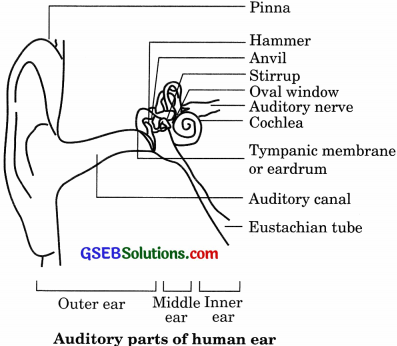# GSEB Solutions Class 9 Science Chapter 12 Sound

Gujarat Board GSEB Textbook Solutions Class 9 Science Chapter 12 Sound Textbook Questions and Answers, Notes Pdf.

## Gujarat Board Textbook Solutions Class 9 Science Chapter 12 Sound

### Gujarat Board Class 9 Science Sound InText Questions and Answers

Page 162

Question 1.
How does the sound produced by a vibrating object in a medium reach your ear?
When an object vibrates, it forces the neighbouring particles of the medium to vibrate. In this way,
vibrations produced by an object are transferred from one particle to another till it reaches the ear.Page 163

Question 1.
Explain how sound is produced by your school bell.
When the school bell is struck with a hammer, it starts vibrating and as a result of the vibration sound waves are produced.

Question 2.
Why are sound waves called mechanical waves?
Waves which need a material medium for propagation are called mechanical waves. Since sound waves also need a material medium for propagation, these are called mechanical waves.

Question 3.
Suppose you and your friend are on the moon. Will you be able to hear any sound produced by your friend?
Sound needs a material medium for their propagation. Since there is no atmosphere on the moon, a person cannot hear the sound produced by another person on it.Page 166

Question 1.
Which wave property determines
(a) loudness
(b) pitch?
(a) The amplitude of the wave determines loudness.
(b) Frequency of the wave determines pitch.

Question 2.
Guess which sound has a higher pitch: guitar or car horn?
A guitar has a higher pitch than a car horn, provided the guitar is properly tuned.

Page 166

Question 1.
What are the wavelength, frequency, time period and amplitude of a sound wave?
For longitudinal wave:

• Wavelength: The distance between two consecutive compressions or two consecutive rarefactions is called the wavelength.
• Frequency: The frequency of a wave is defined as the number of waves produced per second.
• Time period: The time taken by two consecutive compressions or rarefactions to cross a fixed point is called the time period of the wave.
• The amplitude of wave: The magnitude of maximum disturbance on either side of the mean value is called the amplitude of the wave.

Question 2.
How are the wavelength and frequency of sound wave related to its speed?
Speed of sound = frequency x wavelengthQuestion 3.
Calculate the wavelength of a sound wave whose frequency is 220 Hz and speed is 440 m/s in a given medium.
Given,
Frequency (u) = 220 Hz,
Speed (υ) = 440 m/s
Wavelength = $$\frac {u}{υ}$$ = $$\frac {440}{220}$$

Question 4.
A person is listening to a tone of 500 Hz sitting at a distance of 450 m from the source of the sound. What is the time interval between successive compression from the source?
Given,
u = 500 Hz
Time interval T= $$\frac {1}{υ}$$ = $$\frac {1}{500}$$ = 0.002 s.

Page 166

Question 1.
Distinguish between loudness and intensity of sound.
Loudness:
The sensation produced in the ear which enables us to distinguish between a loud and a faint sound is called loudness.
Intensity: The intensity of sound at any point in space is defined as the amount of the energy passing per unit time per unit area in the direction perpendicular to the area.

Page 167

Question 1.
In which of the three media air, water or iron, does sound travel the fastest at a particular temperature?
Sound travels fastest in iron as it is a solid medium.Page 168

Question 1.
An echo was heard after 3 s. What is the distance of the reflecting surface from the source, given that the speed of sound is 342 m/s?
Given,
time (t) = 3 s,
speed (u) = 342 m/s
Distance = $$\frac {υ × t}{2}$$ = $$\frac {342 × 3}{2}$$ = 513 m

Page 169

Question 1.
Why are the ceilings of concert halls curved?
The ceilings of concert halls are curved so that sound after reflection reaches all the corners of the hail.

Page 170

Question 1.
What is the audible range of the average human ear?
20 Hz to 20,000 Hz.

Question 2.
What is the range of frequencies associated with
(a) Infrasound
(b) Ultrasound?
(a) Infrasound: Frequency of less than 20 Hz.
(b) Ultrasound: Frequency lies between 20 Hz and 20 KHz.

Page 172

Question 1.
A submarine emits a sonar pulse, which returns from an underwater cliff in 1.02 s. If the speed of sound in salt water is 1531 m/s, how far away is the cliff?
Given,
time (t) = 1.02 s
speed (u) = 1531 m/s
Distance = $$\frac {u × t}{2}$$ = $$\frac {1531 × 1.02}{2}$$ = 780.81 m.In-Text Activities Solved

(Textbook Page 160)

Activity 12.1

Discussion and conclusion

• A vibrating object produces sound on bringing the vibrating tuning fork near the ear, a sound is heard.
•  On touching one of the prongs of a tuning fork, vibrating sensation is felt.
• When the table tennis ball is gently touched with the prong of the tuning fork, the ball is set into vibration as some energy from tuning fork is transferred to the ball.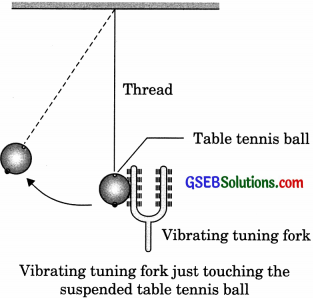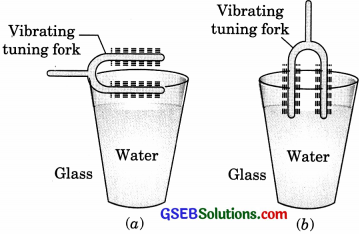Discussion and conclusion

• After touching the water surface with one of the prongs water starts vibrating and waves are produced.
• When both the prongs of the tuning fork are dipped in water in the glass, water splashes out of glass. The energy of a vibrating tuning fork is converted into kinetic energy of water which splashes out of the glass.

(Textbook Page 167)

Activity 12.5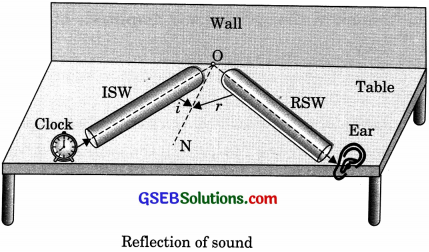Discussion and conclusion
Here angles of incidence is equal to the angle of reflection. The incident sound reflected sound and normal lies in the same plane. Both the first and second law of reflection of sound is satisfied.

### Gujarat Board Class 9 Science Sound Textbook Questions and Answers

Question 1.
What is sound and how is it produced?
A sound is a form of energy and it is produced due to vibrations of different types of object.

Question 2.
Describe with the help of a diagram, how compressions and rarefactions are produced in air near a source of the sound.
When a vibrating object moves forward in air, it pushes and compresses the air in front of it creating a region of high pressure. This region is called a compression (C), as shown in the figure below. The compression starts to move away from the vibrating object.

When the vibrating object moves backwards, it creates a region of low pressure called rarefaction (R), as shown. As the object moves back and forth rapidly, a series of compressions and rarefactions is created in the air. These make the sound wave that propagates through the medium.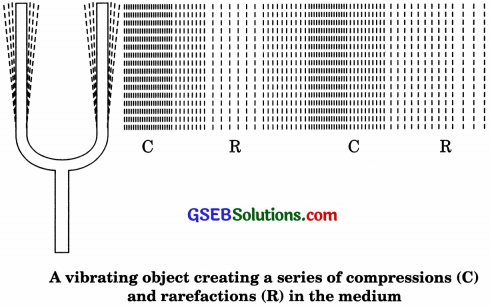Questing 3.
Cite an experiment to show that sound needs a material medium for its propagation.
Take an electric bell and hang this bell inside an empty bell-jar fitted with a Switch vacuum pump. Initially, one can hear the sound of Bell jar ringing bell. Now, pump out some air Electronic bell from the bell-jar using the vacuum pump. It will be observed that the sound of the ringing bell decreases.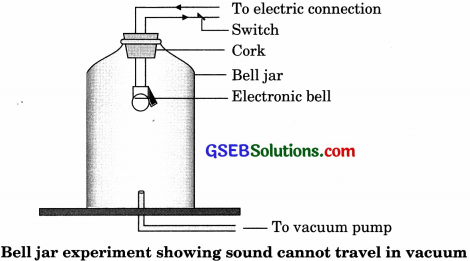If one keeps on pumping the air out of the bell-jar, then at one point, the glass jar will be devoid of any air. Bell jar experiment showing sound cannot travel in a vacuum this moment, no sound can be heard from the ringing bell although one can see that a vacuum is produced. Sound cannot travel through a vacuum. This shows that sound needs a material medium for its propagation.Question 4.
Why is a sound wave called a longitudinal wave?
The vibration of the medium that travels along or parallels to the direction of the wave is called a longitudinal wave.

Question 5.
Which characteristic of the sound helps you to identify your friend by his voice while sitting with others in a dark room?
The quality (or timber) of sound is that characteristic which enables us to distinguish one sound from the other even when these are of the same pitch and loudness.

Question 6.
Flash and thunder are produced simultaneously. But thunder is heard a few seconds after flash is seen, why?
The speed of light is greater than the speed of sound. Thus, the flash of light is seen earlier than the thunder of sound even though both are produced simultaneously.

Question 12.
Give two practical applications of reflection of sound waves.

• Reflection of sound is used to measure the distance and speed of underwater objects. This method is known as Sonar.
• Working of a stethoscope is also based on the reflection of sound. In a stethoscope, the sound of the patient’s heartbeat reaches the doctor’s ear by multiple reflections of sound.

Question 13.
A stone is dropped from the top of a tower 500 m high into a pond of water at the base of the tower. When is the splash heard at the top?
Given, g = 10 m/s2 and speed of sound = 340 m/s.
Given,
Height of tower, s = 500 m
speed of sound, u = 340 m/s
g = 10 m/s2
Initial velocity of the stone, u = 0.
Let time taken by stone to fall to base of tower = t1
Applying, s = ut1 +$$\frac {1}{2}$$ at21
500 = $$\frac {1}{2}$$ x 10 x t21
∴ t1 = 10 s
Now, time taken by the sound to reach the top from the base of tower, t2 = $$\frac {500}{340}$$ =1.47
∴ t2 = 1.47 s
Therefore, the splash is heard at the top after time, t
where t = t1 + t2 = 10 + 1.47= 11.47 s.Question 14.
A sound wave travels at a speed of 339 m/s. If its wavelengths is 1.5 cm, what is the frequency of the wave? Will it be audible?
Speed of sound, υ = 339 m/s
Wavelength of sound wave,
π = 1.5cm = 1.5 x 10-2m
Frequency of sound wave, v = $$\frac{v}{\lambda}=\frac{339}{1.5 \times 10^{-2}}$$ = 22600 Hz
The frequency range of audible sound for human lies between 20 Hz and 20,000 Hz. Since the frequency of the given sound is more than 20,000 it is not audible.

Question 15.
What is reverberation? How can it be reduced?
A sound created in a big hail will persist by repeated reflection from the walls until it is reduced to a value where it is no longer audible. The repeated reflection that results in the persistence of sound is called reverberation.

Question 16.
What is the loudness of sound? What factor does it depend on?
A loud sound has high energy. Loudness depends on the amplitude of vibrations. In fact, loudness is proportional to the square of the amplitude of vibrations.

Question 17.
Explain how bats use ultrasound to catch prey.
The ultrasonic waves emitted by the bat are detected by its ears. The nature of reflected waves tells the bat about the location and the nature of its prey.Question 18.
How is ultrasound used for cleaning?
Objects to be cleaned are put in a cleaning solution and ultrasound waves are passed through that solution. The high frequency of these ultrasound waves detaches the dirt from the objects.

Question 19.
Explain the working and application of a sonar.
The acronym Sonar stands for Sound Navigation and Ranging.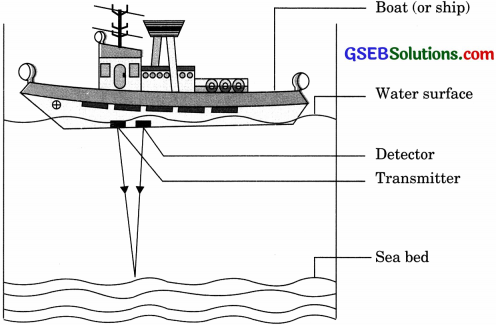Ultrasound sent by the transmitter and received by the detector

1. Sonar is a device that uses ultrasonic waves to measure the distance, direction and speed of underwater objects.
2. Sonar consists of a transmitter and a detector and is installed in a boat or a ship.
3. The transmitter produces and transmits ultrasonic waves. These waves travel through water and after striking the object on the seabed, get reflectéd back and are sensed by the detector.
4. The detector converts the ultrasonic waves into electrical signals which are appropriately interpreted.
5. The distances of the object that reflected the sound wave can be calculated by knowing the speed of sound in water and the time interval between transmission and reception of the ultrasound.
6. Let the time interval between transmission and reception of ultrasound signal be t and the speed of sound through seawater be y.
7. The total distance, 2d travelled by the ultrasound is then, 2d = u x t.
8. The above method is called echo-ranging. The sonar technique is used to determine the depth of the sea and to locate underwater hills, valleys, submarine, icebergs, a sunken ship, etc.

Question 20.
A sonar device on a submarine sends out a signal and receives an echo 5 s later. Calculate the speed of sound in water if the distance of the object from the submarine is 3625 m.
Time is taken to hear the echo, t = 5 s
The distance of the object from the submarine,
d = 3625 m
Total distance travelled by the sonar waves during the transmission and reception in water = 2d
Velocity of sound in water, v =$$\frac{2 d}{t}=\frac{2 \times 3625}{5}$$ = 1450 ms-1Question 21.
Explain how defects in a metal block can be detected using ultrasound.
Defects in the metal block do not allow ultrasound to pass through them and they are reflected back. This fact is used to detect defects in metal blocks. Ultrasound is passed through one end of a metal block and detectors are placed on the other end. The defective part of the metal block does not allow ultrasound to pass through it. As a result, it will not be detected by the detector. Hence, defects in metal blocks can be detected using ultrasound.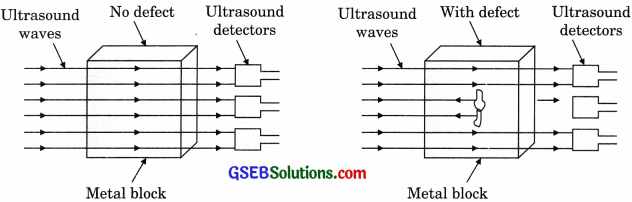Question 22.
Explain how the human ear works.